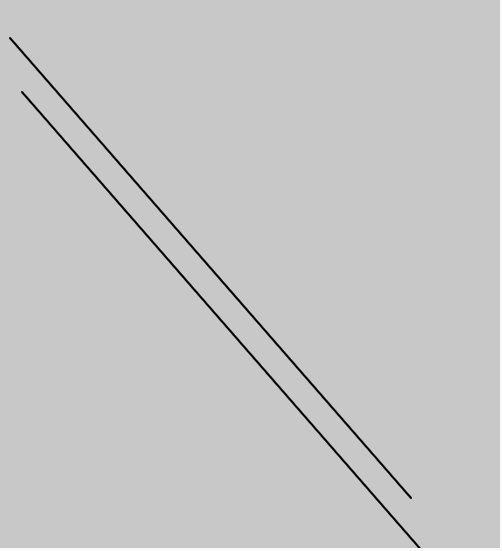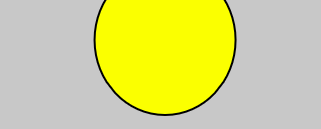p5.js | createVector() function

• Last Updated : 05 Oct, 2021

The createVector() function in p5.js is used to create the new p5 vector which contains both magnitude and direction. This provides a two or three-dimensional vector, specifically a geometric vector.

Syntax:

createVector([x], [y], [z])

Parameters: This function accepts three parameters as mentioned above and described below:

• x: This parameter stores the x component of the vector.
• y: This parameter stores the y component of the vector.
• z: This parameter stores the z component of the vector.

Below programs illustrate the createVector() function in p5.js:

Example 1: This example uses createVector() function to draw a line.

Javascript

 function setup() {     // Create a Canvas    createCanvas(500, 550);} function draw() {        // Vector initialisation    // using createVector    t1 = createVector(10, 40);    t2 = createVector(411, 500);        // Set background color     background(200);        // Set stroke weight    strokeWeight(2);        // line using vector    line(t1.x, t1.y, t2.x, t2.y);        translate(12, 54);      line(t1.x, t1.y, t2.x, t2.y);}

Output:Example 2: This example uses createVector() function to draw a circle.

Javascript

 function setup() {     // Create a Canvas    createCanvas(500, 550);}  function draw() {        // Vector initialisation    // using createVector    t1 = createVector(10, 40);    t2 = createVector(41, 50);        // Set background color     background(200);        // Set stroke weight    strokeWeight(2);        // Fill yellow    fill('yellow');        // ellipse using vector    ellipse(t1.x*millis() / 1000 * 20,            t1.y, t2.x+100, t2.y+100);}

Output:Hey geek! The constant emerging technologies in the world of web development always keeps the excitement for this subject through the roof. But before you tackle the big projects, we suggest you start by learning the basics. Kickstart your web development journey by learning JS concepts with our JavaScript Course. Now at it's lowest price ever!

My Personal Notes arrow_drop_up